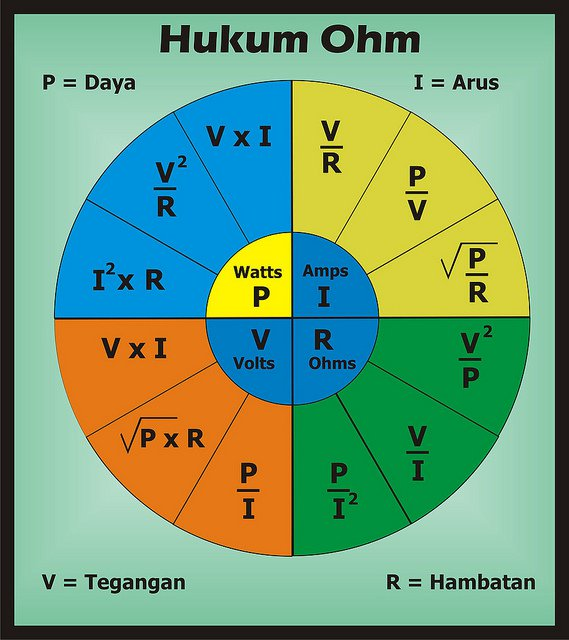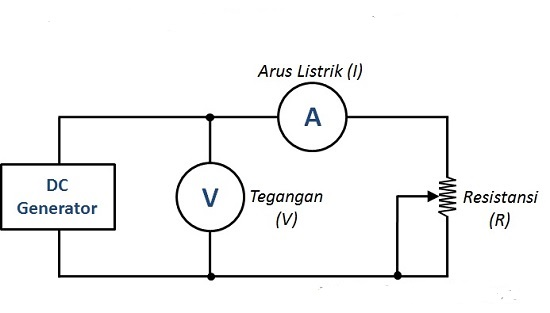Breaking News
Home / Computer / Formula, Sound, Sample Problem + Discussion [LENGKAP]# Formula, Sound, Sample Problem + Discussion [LENGKAP]

In a physics or electrical subject, there must be a lesson to discuss about Ohm's law, and do you know what is meant by Ohm's law?

In Ohm's law there is also a sound and Ohm's legal formula. Where the sound and the formula are the most important guidelines in working on the problem and applying Ohm's law in everyday life.

Ohm's law is also a basic law that can express a relationship between voltage (V), electric current (I) and also obstacles (R). In English also Ohm's law is often referred to as Ohm's Laws

For the first time Ohm's law was introduced by a German physicist whose name was Geord Simon Ohm in 1825. Ohm's Law he has included it in a paper which has the title The Galvanic Circuit Investigated Mathematically in 1827.

## Sounds of Ohm's LawBefore entering into sound discussion Ohm's law, you must first understand what the Ohm's Law means.

Ohm's law is a law that states that the magnitude of the current flowing into a conductor will be proportional to the potential difference between the ends of the conductor if the temperature can be maintained it is consistent.

It can also be mentioned that Ohm's law is a statement of the magnitude of the current that will flow through sebu the conductor and is always directly proportional to the potential difference previously set to him.

A conductor can be said to have obeyed the Ohm law, if the value of the resistance does not depend on the size and also the potential difference polarity contained in it.  Even though the above statements do not apply to all types of conductors, the term of said law is still used.

After understanding the meaning of Ohm's law, below will explain what sounds from Ohm's law. Next Sounds of Ohm's Law:## Ohm's Law FormulaOhm's Law can be mathematically formulated as in the following equation: V = I x R

I = V / R

R = V / I

Description:

V = is a Voltage (Differential Potential or Voltage whose unit is Volt (V))
I = is a Current (Electric Current whose unit is Ampere (A))
R = is a Resistance (Barriers or Resistance whose unit is Ohm ()))

In its application, you can use an Ohm Law Theory in Electronic Circuits to be able to reduce voltage, reduce electric current, and it can also be able to obtain the hanbatan value commonly referred to as the resistance you want.

One of the things you must remember is always in calculating the formula for an Ohm law, units that can be used namely Ampere, Ohm and also Volt.  If you are use other units, for example, such as miliampere, millivolt, kilovolt, kiloohm, and megaohm, so you also have to convert into Ampere units, Ohm or Volt beforehand, you can make calculations easier and you can also get the correct results.  Examples of Ohm Law Questions + Answers

By working on Ohm's law questions, you can improve your abilities and understanding in the subjects of Ohm's law. Here are some examples of Ohm's law problems and their answers.We really need a DC Generator or commonly called a Power Supply, Ammeters, Voltmeter and a Potentiometer that is right with value that will be needed.

### 1. Example Problem for Calculating Electric Current (I)

To solve the problem of calculating an electric current (I), we must use the formula I = V x R .

Example Example:

Arrange the power supply or DC generator so that it can produce output from a voltage of 10 V, then also set the value of the Potentiometer to 10 Ohms. And determine what value of electric current (I)!

To be able to solve the above problem, we must enter a voltage value that has a number of 10 V and also the resistance value of the potentiometer the number of 10 Ohms to the Ohm law formula, as in the following:

I = V / R
I = 10/10
I = 1 Ampere

And it can be concluded that the value I, namely 1 Ampere .

### 2. Problem Example for Calculating V (Voltage)

To solve a problem of calculating voltage (V) or potential difference, we must use the formula V = I x R .

Problem Example:

Set the value of the resistance (R) or Potentiometer resistance to 500 Ohm, and then set the DC Generator or Power supply until you get an Electric Current (I) of 10 mA. And calculate the voltage he gets!

To work on the above problem, we need to first converge the unit of Electricity represented by I 1945 which is still millimeter , and turn it into an Ampere unit, namely: 10 mA – 0.01 Ampere

Next enter the value of the potentiometer resistance which amounts to 500 Ohm and the value of Electric Current (I). ] 0.01 Ampere into the Ohm legal formula as follows:

V = I x R
V = 0.01 x 500
V = 5 Volt

And it can be concluded that the value of V is 5 Volt .

### 3. Problem Example for Calculating R (Barriers or Resistance)

To solve the problem of calculating resistance or resistance (R), we must use the formula R = V x I .

Example Problem:

If the value of the voltage on the Volmeter is 12 V and the value of the electric current in the Ammeters unit is 0.5 A. Determine how many nilations of the Potentiometer's Resistance! To solve the above problem, it is necessary to enter the value of the voltage of 12 V and the electric current value of 0.5 A in the Ohm formula, as follows:

R = V / I
R = 12 / 0.5.51919909037 ] R = 24 Ohm

And it can be concluded that the value of R is 24 Ohm . along with the discussion. Hopefully useful and easy to understand. Thank you!